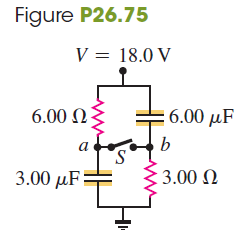# Problem: (a) What is the potential of point a with respect to point b in Fig. P26.75 when the switch S is open? (b) Which point, a or b, is at the higher potential? (c) What is the final potential of point b with respect to ground when S is closed? (d) How much does the charge on each capacitor change when S is closed?

###### FREE Expert Solution

The charge is expressed as:

$\overline{){\mathbf{Q}}{\mathbf{=}}{\mathbf{C}}{\mathbf{V}}}$, where C is capacitance and V is the voltage.

(a)

When S is open, after the equilibrium, the capacitors get fully charged.

There will be no current flowing through the capacitors.

Therefore, the force across each capacitor is the voltage across the battery.

Also, the voltage across the resistors should be zero when S is open.

The voltage across the battery is 18.0 V.

99% (473 ratings)###### Problem Details

(a) What is the potential of point a with respect to point b in Fig. P26.75 when the switch S is open?

(b) Which point, a or b, is at the higher potential?

(c) What is the final potential of point b with respect to ground when S is closed?

(d) How much does the charge on each capacitor change when S is closed?